Previous Year Questions: Introduction to Trigonometry

# Previous Year Questions: Introduction to Trigonometry - Mathematics (Maths) Class 10

Q1: sin2 60° + 2 tan 45° - cos2 30°    [Allahabad 2019]
Ans:  2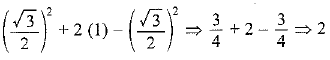Q2: If sin x + cos y = 1; x = 30° and is an acute angle, find the value of y.  [CBSE 2019 (30/5/1)]
Ans:
We have, sin x + cosy = 1
⇒ sin30° + cosy = 1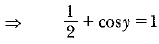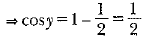⇒ y = 600

Q3: Prove that: (1 + cot A - cosec A)(l + tan A + sec A) = 2   [CBSE 2019 (30/1/2)]
Ans:
LHS = (1 + cotA-cosecA) (1 + tanA +secA)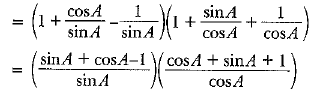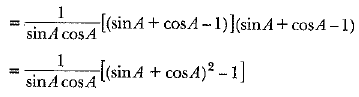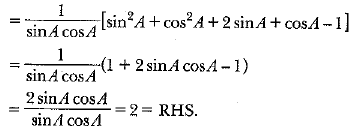Q4: Prove that:        [CBSE 2019 (30/5/1)]
Ans: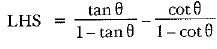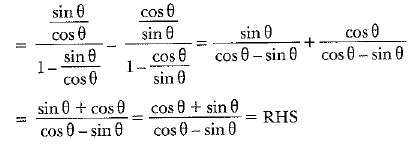Q 5: If cos θ + sin θ = √2cos θ , show that cos θ - sin θ = √2 sin θ.  [CBSE 2019 (30/ 5/ 1)]
Ans: Given: cos θ + sin θ = √2cos θ

Now, (cos θ + sin θ)2 + ( cos θ - sin θ)2
cos2θ + sin2θ + 2cosθ. sin θ + cos2θ + sin2θ - 2cosθ. sinθ
1 + 1 = 2

Again, (cos θ + sin θ)2 + (cos θ - sin θ)2 = 2
⇒ (√2 cosθ)2 + (cos θ - sin θ)2 = 2
⇒ 2 cos2θ + ( cos θ - sin θ)2 = 2
⇒ (cos θ - sin θ)2 = 2 - 2cos2θ = 2 ( 1 - cos2θ)
= 2sin2θ
∴ cos θ - sin θ = √2 sin θ
Hence proved

Q6: Prove that: ( sin A + cosec A)2 + ( cos A + sec A)2 = 7 + tan2 A + cot2 4

[CBSE 2019 (30/1/2;]

Ans: LHS = (sin A + cosec A)2 + (cos A + sec A)2
=sin2 A + cosec2 A + 2sinA . cosec A + cos2 A + sec2 A + 2 cos A .sec A
= (sin2 A + cosec2 A + 2) + (cos2 A + sec2 A + 2)     (sin A.cosec A = 1)
= (sin2 A + cos2A) + (cosec2 A + sec2 A) + 4      (cos A. sec A = 1)
= 1 + 1+ cot2 A + 1 + tan2 A + 4
= 7 + tan2 A + cot2 A = RHS (∵ 1 + cot2 A = cosec2 A and 1 + tan2 A = sec2 A)

Q7: If sin (A + 2B)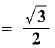and cos (A + 4B) = 0, A > B, and A + 4B ≤ 900 then find A and B. [CBSE 2018 (C)]
Ans: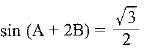So, sin(A + 2B) = sin 60°
Hence A + 2B = 60°     ..(i)
Also, we have
cos(A + 4B) = 0
cos(A + 4B) = cos 90°
A + 4B = 90°     ..(ii)
Subtracting (ii) from (i), we have
-2B = - 30°
=> B = 15°
Put B = 15° in eq. (i),  we have
A + 2(15°) = 60°
⇒ A + 30° = 60°
⇒ A = 30°

Q1: Prove that: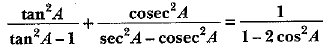[CBSE 2019 (30/5/1)]
Ans: LHS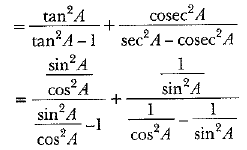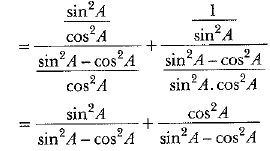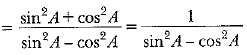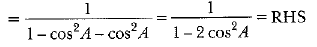Q2: Prove that: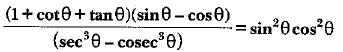[CBSE 2019 (30/5/1)]
Ans: LHS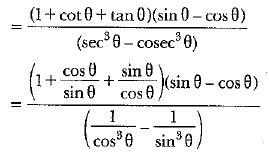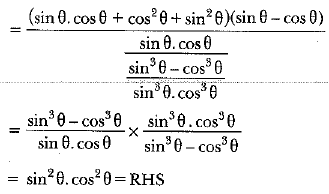The document Previous Year Questions: Introduction to Trigonometry | Mathematics (Maths) Class 10 is a part of the Class 10 Course Mathematics (Maths) Class 10.
All you need of Class 10 at this link: Class 10

## Mathematics (Maths) Class 10

115 videos|478 docs|129 tests

## FAQs on Previous Year Questions: Introduction to Trigonometry - Mathematics (Maths) Class 10

 1. What is trigonometry and why is it important?Ans. Trigonometry is a branch of mathematics that deals with the relationships between the angles and sides of triangles. It is important because it has numerous applications in various fields such as physics, engineering, and navigation. Trigonometry allows us to solve problems involving distances, heights, and angles, making it essential in real-world scenarios.
 2. What are the basic trigonometric ratios and how are they used?Ans. The basic trigonometric ratios are sine, cosine, and tangent, denoted as sin, cos, and tan respectively. These ratios relate the angles of a right triangle to the lengths of its sides. - Sine (sin) is the ratio of the length of the side opposite the angle to the hypotenuse. - Cosine (cos) is the ratio of the length of the adjacent side to the hypotenuse. - Tangent (tan) is the ratio of the length of the side opposite the angle to the adjacent side. These ratios are used to find missing side lengths or angles in a right triangle, using the given information.
 3. How do we find the values of trigonometric ratios for different angles?Ans. The values of trigonometric ratios for different angles can be found using a trigonometric table or a scientific calculator. However, it is important to note that these values are typically given for specific angles, such as 0°, 30°, 45°, 60°, and 90°. For other angles, we can use the concept of periodicity to find their values. The values of trigonometric ratios repeat in a cyclical pattern every 360° (or 2π radians), so we can use the known values for these special angles and apply the properties of periodicity to find the values for other angles.
 4. How is trigonometry used in real-world applications?Ans. Trigonometry is used in various real-world applications, including: - Architecture: Trigonometry is used to calculate angles and distances in architectural designs, ensuring the structural integrity of buildings. - Navigation: Trigonometry is used in navigation systems, such as GPS, to determine the position, direction, and distance between two points. - Engineering: Trigonometry is used in engineering fields, such as civil and mechanical engineering, to design and construct structures and machines. - Astronomy: Trigonometry is used in astronomy to calculate the positions and movements of celestial bodies. - Physics: Trigonometry is used in physics to analyze the motion of objects, such as projectiles, and to calculate forces and vectors.
 5. How can trigonometry help in solving problems involving heights and distances?Ans. Trigonometry is particularly useful in solving problems involving heights and distances. By using the principles of trigonometry, we can calculate the height or distance of an object without physically measuring it. For example, if we know the angle of elevation to an object and the distance from the observer to the object, we can use trigonometry to calculate the height of the object. Similarly, if we know the angles of elevation or depression and the distance between two points, we can use trigonometry to calculate the vertical or horizontal distance between the points. This makes trigonometry a valuable tool in fields such as surveying, construction, and architecture.

## Mathematics (Maths) Class 10

115 videos|478 docs|129 testsExplore Courses for Class 10 examSignup to see your scores go up within 7 days! Learn & Practice with 1000+ FREE Notes, Videos & Tests.
10M+ students study on EduRev
Track your progress, build streaks, highlight & save important lessons and more!
Related Searches

,

,

,

,

,

,

,

,

,

,

,

,

,

,

,

,

,

,

,

,

,

;